# Bounds of the remainder of a Taylor series

• songoku
By both sides of ##a=1##, do you...mean check on both sides of a=1 or check on both sides of x'=1?Checking on both sides of a=1.

#### songoku

Homework Statement
Given that ##f(x)=\frac{1}{1+x}##. Find bound of remainder ##R_3 (x)## assuming ##|x-1| \leq2##
Relevant Equations
Taylor series

Taylor Inequality
I have found the Taylor series up to 4th derivative:
$$f(x)=\frac{1}{2}-\frac{1}{4}(x-1)+\frac{1}{8}(x-1)^2-\frac{1}{16}(x-1)^3+\frac{1}{32}(x-1)^4$$

Using Taylor Inequality: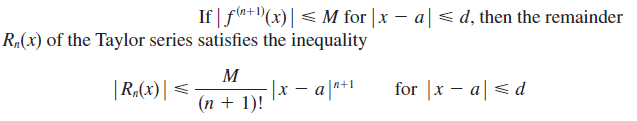##a=1, d=2## and ##f^{4} (x)=\frac{24}{(1+x)^5}##

I need to find M that satisfies ##|f^4 (x)| \leq M##

From ##|x-1| \leq 2 \rightarrow 0 \leq x+1 \leq 4## , then ##0 \leq (x+1)^5 \leq 4^5##

Putting the boundary of ##(1+x)^5## to ##f^{4} (x)##, there is no upper boundary of ##f^{4} (x)## which does not make sense. And actually ##x+1## can not be zero because ##f^{4} (x)## would be undefined so my approach is certainly wrong.

How to find M? Thanks

Last edited:
For any given ##x : |x+1|\le 2## you can get a bound that is finite.
CORRECTION: Should be ##x : |x-1|\le 2##

Last edited:
FactChecker said:
For any given ##x : |x+1|\le 2## you can get a bound that is finite.
I am sorry, but how to get ##|x+1|\le 2##?

Thanks

songoku said:
Putting the boundary of ##(1+x)^5## to ##f^{4} (x)##, there is no upper boundary of ##f^{4} (x)## which does not make sense. And actually ##x+1## can not be zero because ##f^{4} (x)## would be undefined so my approach is certainly wrong.
It actually does make sense that you can't find a bound since the function ##\frac 1{1+x}## blows up as ##x \to -1##.

•songoku
vela said:
It actually does make sense that you can't find a bound since the function ##\frac 1{1+x}## blows up as ##x \to -1##.
So the answer the question is: no bound for ##R_3 (x)##?

Thanks

songoku said:
I am sorry, but how to get ##|x+1|\le 2##?

Thanks
Sorry. I should have said ##|x-1|\le 2##, which is given in the problem statement. I will edit my post.

songoku said:
So the answer the question is: no bound for ##R_3 (x)##?
There's no bound on the interval ##-1 \le x \le 3##, but is that the interval relevant for calculating the remainder?

•songoku and FactChecker
vela said:
There's no bound on the interval ##-1 \le x \le 3##, but is that the interval relevant for calculating the remainder?
Sorry, that's the only interval I can get. I also have checked the interval of convergence, which is ##-1 < x < 3## and still get no upper bound for ##R_3 (x)##. I can't find any other interval related to the question

songoku said:
Sorry, that's the only interval I can get. I also have checked the interval of convergence, which is ##-1 < x < 3## and still get no upper bound for ##R_3 (x)##. I can't find any other interval related to the question
For any particular value ## x'## in (-1,3) we do not care about the entire interval [-1,3]. We only care about ##|x-1|\le |x'-1|##. The function derivatives are bounded in that.

•songoku
FactChecker said:
For any particular value ## x'## in (-1,3) we do not care about the entire interval [-1,3]. We only care about ##|x-1|\le |x'-1|##. The function derivatives are bounded in that.
I draw graph of ##\frac{24}{(1+x)^5}##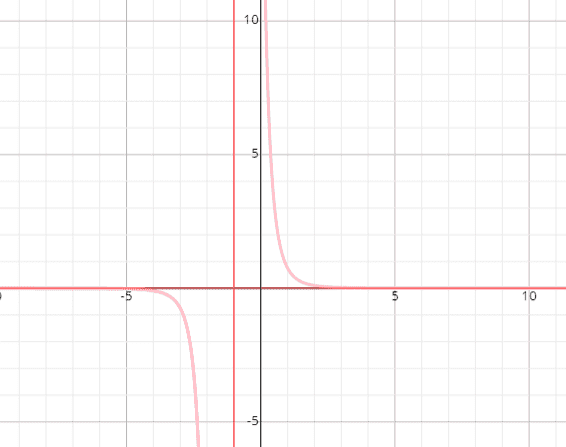It is monotonically decreasing in interval ##-1<x<3## and the range will be ##(\frac{3}{128}, \infty )##

I also draw the graph of ##|x-1|##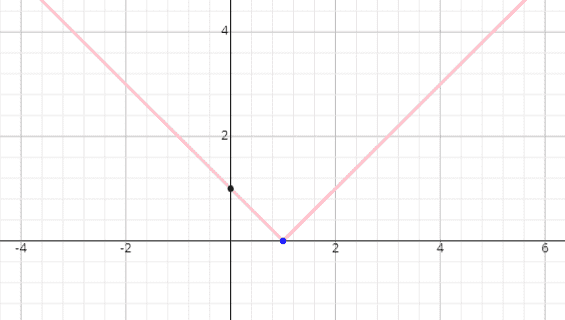But sorry I don't understand how to find the interval where ##|x-1| \le |x'-1|##

songoku said:
But sorry I don't understand how to find the interval where ##|x-1| \le |x'-1|##
When trying to bound the error of the partial series at a point ##x'##, it is only necessary to look at the interval of points ##x## that are at least as close to the 1 as ##x'## is. That is for x in the interval [1-|x'-1|, 1+|x'-1|].

•songoku
FactChecker said:
When trying to bound the error of the partial series at a point ##x'##, it is only necessary to look at the interval of points ##x## that are at least as close to the 1 as ##x'## is. That is for x in the interval [1-|x'-1|, 1+|x'-1|].
So I need to find ##M## where ##|f^{3} (x')| \le M## and ##x' \in [a,x]##

##a \le x' \le x \rightarrow 1 \le x' \le 3## so the value of M is ##\frac{3}{4}##

Is this correct? Thanks

songoku said:
So I need to find ##M## where ##|f^{3} (x')| \le M## and ##x' \in [a,x]##

##a \le x' \le x \rightarrow 1 \le x' \le 3## so the value of M is ##\frac{3}{4}##

Is this correct? Thanks
You need to check on both sides of a=1, not just the side that x' is on. I think that we are using different notations, but the idea seems right.

FactChecker said:
I think that we are using different notations
I also think so. Honestly I am still not really understand the notation ##|x-1| \le |x'-1|##. I only use Taylor inequality I posted in #1 but after you introduced ##x'##, I searched through the web and found this link:

https://brilliant.org/wiki/taylor-series-error-bounds/

This is part of what is in there: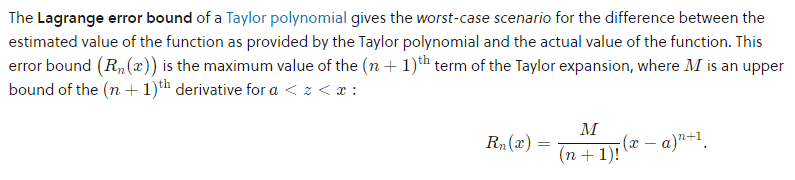and also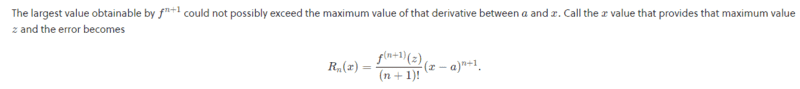At first, I thought ##x## in ##f^{n+1} (x)## is exactly the same as ##x## in ##|x-a| \le d## but in that link, it is written as ##f^{n+1} (z)## instead where ##a<z<x## so I thought ##a## will always be the lower boundary of ##z##, that's why I only checked the value bigger than 1.

FactChecker said:
You need to check on both sides of a=1, not just the side that x' is on.
By both sides of ##a=1##, do you mean for 2 intervals, something like ##p<x'<1## and ##1<x'<3## where ##p## is a value that I need to find first?

Thanks

FactChecker said:
You need to check on both sides of a=1, not just the side that x' is on.
$$R_n(x) = \frac{f^{(n+1)}(c)}{(n+1)!}(x-a)^{n+1},$$ where ##c## is between ##a## and ##x##. It seems as long as you find an upper bound ##M## for ##f^{(n+1)}(c)## on ##(a,x)## (or ##(x,a)## if ##x<a##), you should be fine.

songoku said:
I also think so. Honestly I am still not really understand the notation ##|x-1| \le |x'-1|##.
Take ##x'## as fixed. You're finding an upper bound for ##R_n(x)## in the interval ##|x-a|<d## with ##d = |x'-a|##.

•songoku
vela said:
$$R_n(x) = \frac{f^{(n+1)}(c)}{(n+1)!}(x-a)^{n+1},$$ where ##c## is between ##a## and ##x##. It seems as long as you find an upper bound ##M## for ##f^{(n+1)}(c)## on ##(a,x)## (or ##(x,a)## if ##x<a##), you should be fine.

Take ##x'## as fixed. You're finding an upper bound for ##R_n(x)## in the interval ##|x-a|<d## with ##d = |x'-a|##.
You are proposing that there is a stronger theorem about the bound on the error. I am not aware of it.
CORRECTION: Apparently there is a stronger theorem. It is referenced in @songoku 's post #14)

vela said:
Take ##x'## as fixed. You're finding an upper bound for ##R_n(x)## in the interval ##|x-a|<d## with ##d = |x'-a|##.
I will try to use that:

$$d=|x'-a|$$
$$2=|x'-1|$$
$$x'=3 ~\text{or} ~x'=-1$$

Since ##x' \in (a,x)## or ##x' \in (x,a)##, there will be 2 intervals, which are ##-1 < x' < 1## or ##1 < x' < 3##. No upper bound for the remainder in interval ##-1 <x'<1## so the one I should use is ##1<x'<3##

Is this correct? Thanks

Last edited:
I've deleted a couple of my posts and replies to them, as I really misread the graph in post #10. As a result, my responses didn't add anything to the thread.

songoku said:
Is this correct? Thanks
No.

Say you want to find an upper bound for ##R_3(1.5)##, i.e., ##x'=1.5##. The remainder is equal to
$$R_3(1.5) = \frac {f''''(c)}{4!} (1.5-1)^4$$ for some ##c## in the interval ##(1,1.5)##. If you find an upper bound ##M## for ##f''''(x)## on the interval ##(1,1.5)##, then you can say
$$R_3(1.5) \le \frac {M}{4!} (1.5-1)^4.$$ Note that you don't really care that the series converges between ##-1 < x \le 3## to find an upper bound for the remainder ##R_3(1.5)##.

•songoku
vela said:
No.

Say you want to find an upper bound for ##R_3(1.5)##, i.e., ##x'=1.5##. The remainder is equal to
$$R_3(1.5) = \frac {f''''(c)}{4!} (1.5-1)^4$$ for some ##c## in the interval ##(1,1.5)##. If you find an upper bound ##M## for ##f''''(x)## on the interval ##(1,1.5)##, then you can say
$$R_3(1.5) \le \frac {M}{4!} (1.5-1)^4.$$ Note that you don't really care that the series converges between ##-1 < x \le 3## to find an upper bound for the remainder ##R_3(1.5)##.
Let me try again:

I want to find an upper bound for ##R_3(x)##. The remainder is equal to
$$R_3(x) = \frac {f''''(c)}{4!} (x-1)^4$$ for some ##c## in the interval ##(1,x)## or ##(x,1)##. There will be 2 intervals since I do not know whether ##x>1## or ##x<1##

From the question: ##|x-1| \le 2## so ##-1 \le x \le 3##

Then how to proceed to find the relevant interval for the remainder? Thanks

songoku said:
how to proceed to find the relevant interval for the remainder?
If I understand the question, it can be any interval containing x and 1. So for x<1 you can choose [x,1).

Last edited:
•songoku and FactChecker
haruspex said:
If I understand the question, it can be any interval containing x and 1. So for x<1 you can choose [x,1).
So for ##x<1## the interval is ##[x,1)## and for ##x>1## the interval is ##(1,x]##.

Then how to find the upper bound? Thanks

songoku said:
So for ##x<1## the interval is ##[x,1)## and for ##x>1## the interval is ##(1,x]##.

Then how to find the upper bound? Thanks
Is there a local extremum within the interval? What about values at the ends of the interval?

•songoku
haruspex said:
Is there a local extremum within the interval? What about values at the ends of the interval?
Since ##\frac{24}{(1+x)^5}## is monotonically decreasing in interval ##-1<x \le 3##, there is no local extremum so the upper bound will the located at the left end-point of the interval.

I think I only need to find upper bound either on ##[x,1)## or ##(1,x]## so the one I can use is ##(1,x]##. The upper bound will be ##\frac 3 4##

Is this correct? Thanks

songoku said:
Since ##\frac{24}{(1+x)^5}## is monotonically decreasing in interval ##-1<x \le 3##, there is no local extremum so the upper bound will the located at the left end-point of the interval.

I think I only need to find upper bound either on ##[x,1)## or ##(1,x]## so the one I can use is ##(1,x]##. The upper bound will be ##\frac 3 4##

Is this correct? Thanks
You should find a separate bound for the two cases, depending on where x is. And remember that the original problem was to find the bounds, not just the upper bound. So you may need to calculate a maximum and a minimum. (They might all have the same magnitude, but they might not in all example problems.)

•songoku
FactChecker said:
You should find a separate bound for the two cases, depending on where x is. And remember that the original problem was to find the bounds, not just the upper bound. So you may need to calculate a maximum and a minimum. (They might all have the same magnitude, but they might not in all example problems.)
For ##[x,1)## the upper bound will be ##\frac{24}{(1+x)^5}## and lower bound is ##\frac 3 4##

For ##(1,x]## the upper bound will be ##\frac{3}{4}## and lower bound will be ##\frac{24}{(1+x)^5}##

Is this correct? Thanks

Looks ok to me.

•songoku
Thank you very much for the help and explanation FactChecker, vela, Mark44, haruspex